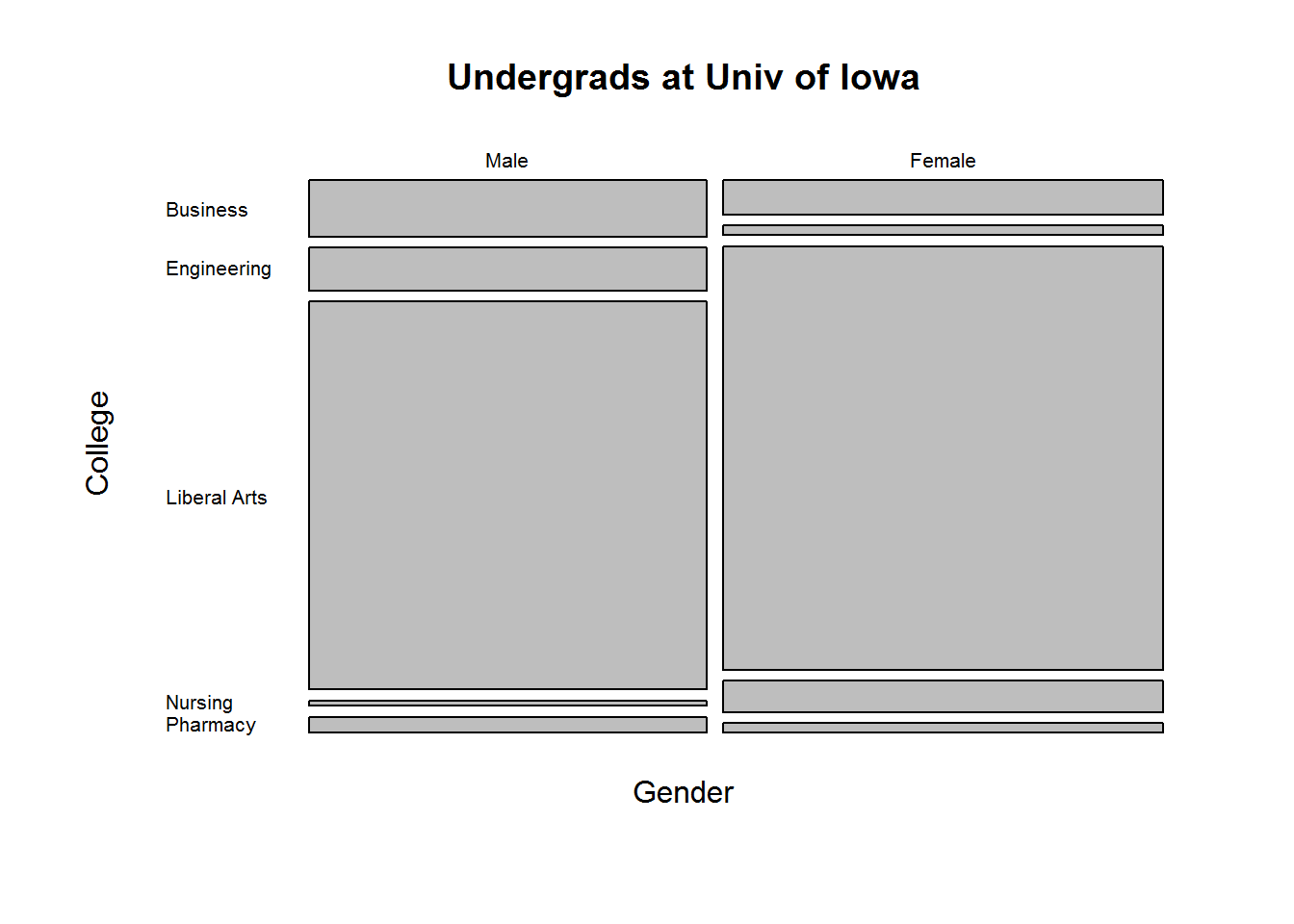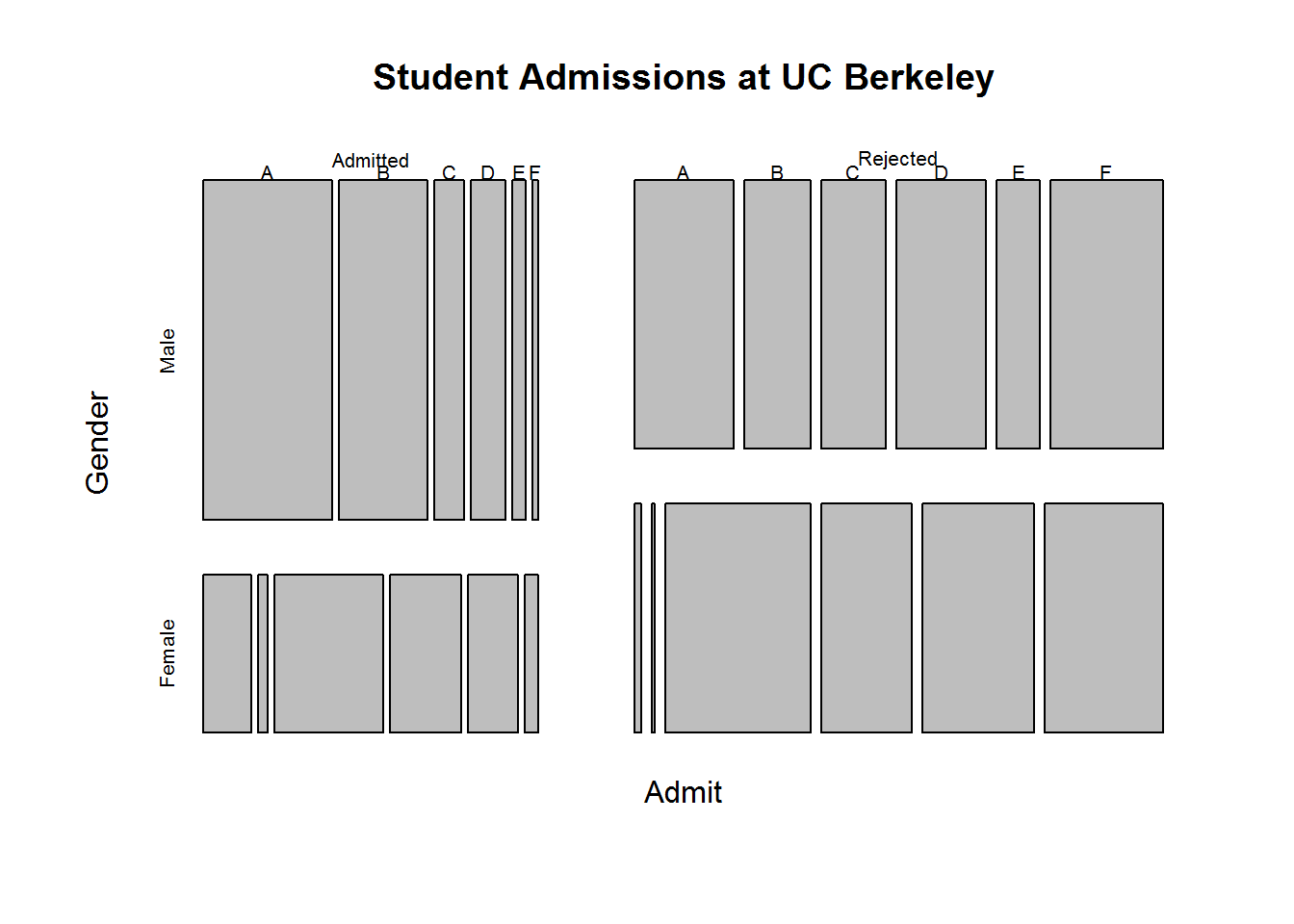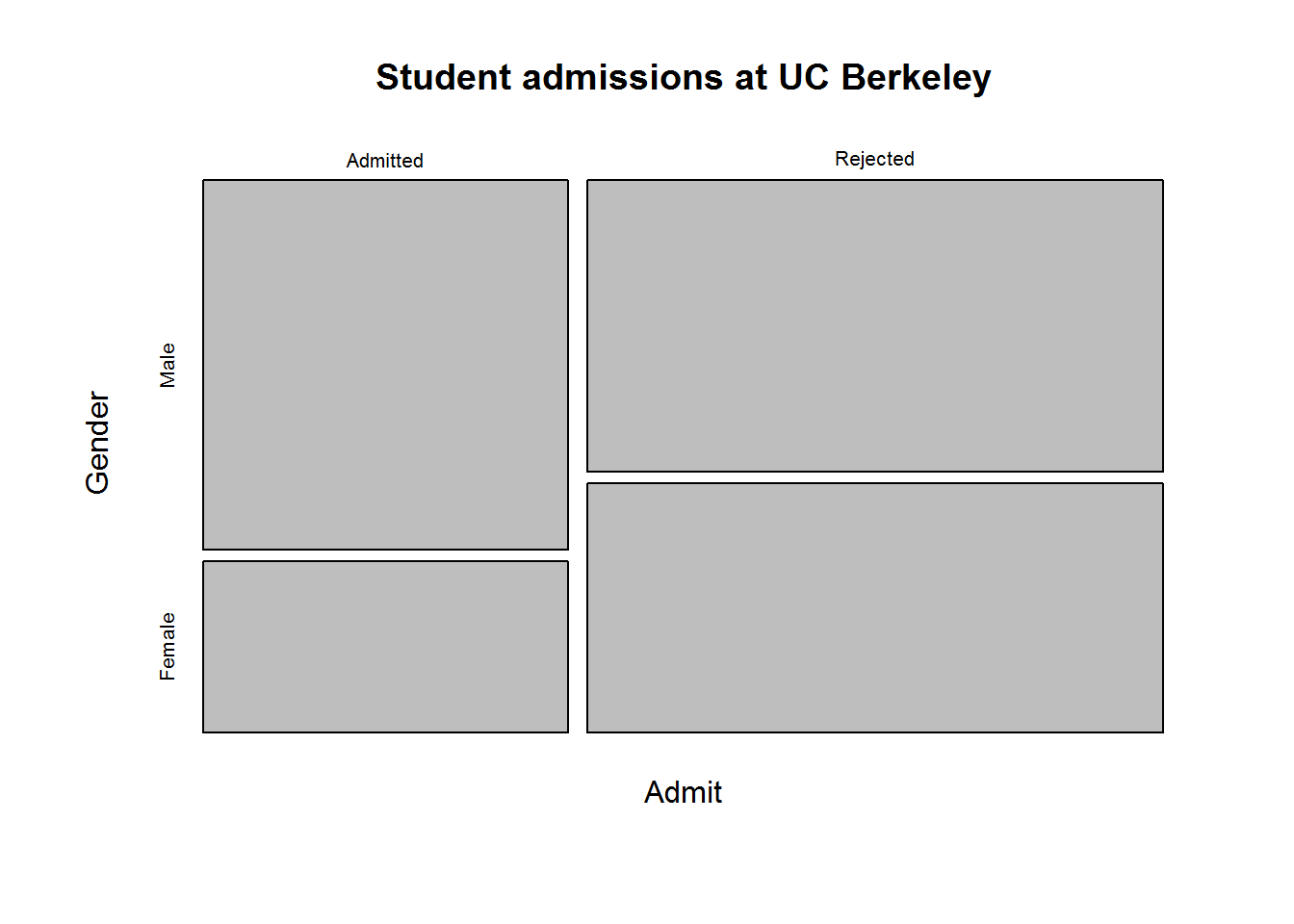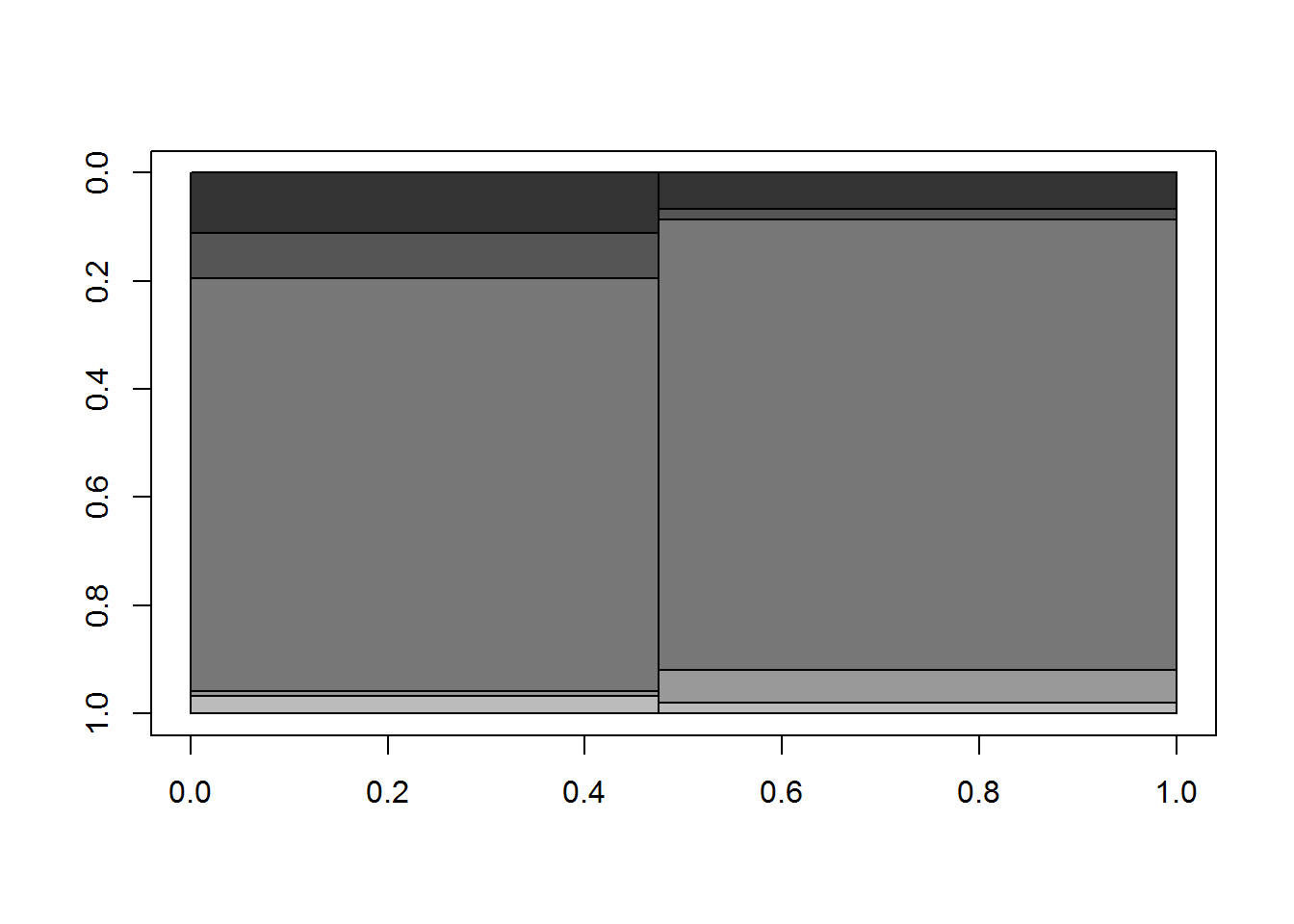# Understanding Mosaic Plots

Mosaic plots provide a way to visualize contingency tables. However they’re not as intuitive as, say, a scatter plot. It’s hard to mentally map a contingency table of raw counts to what you’re seeing in a mosiac plot. Let’s demonstrate.

Here I use data from Example 8.6-3 of Probability and Statistical Inference (Hogg & Tanis, 2006). These data are a random sample of 400 University of Iowa undergraduate students. The students were classified according to gender and the college in which they were enrolled.

M <- matrix(data = c(21,14,16,4,145,175,2,13,6,4),
ncol=5,
dimnames = list(Gender=c("Male","Female"),

M
##         College
## Gender   Business Engineering Liberal Arts Nursing Pharmacy
##   Male         21          16          145       2        6
##   Female       14           4          175      13        4

To create a basic mosaic plot in R, you use the mosaicplot function with a contingency table as the first argument. This can be a table or matrix object. I’ve also added a title to the graph and used the las argument to make the axis labels horizontal.The main feature that likely jumps out to you is the lack of numbers. We just see rectangles stacked on one another. If we compare the mosaic plot to the table of counts, the size of the boxes seem related to the counts in the table. Indeed they are, but how? Another feature you may notice is that the widths of the rectangles do not vary but the heights do. What’s up with that?

By default, the mosaicplot function recursively calculates marginal proportions starting with the rows. In our example, we start with gender:

apply(M, 1, function(x)sum(x)/sum(M))
##   Male Female
##  0.475  0.525

That simply says 0.475 of our sample is male, and 0.525 is female. These are the widths of the rectangles.

Now within gender, calculate the proportion belonging to each college:

prop.table(M, margin = 1)
##         College
## Gender     Business Engineering Liberal Arts    Nursing   Pharmacy
##   Male   0.11052632  0.08421053    0.7631579 0.01052632 0.03157895
##   Female 0.06666667  0.01904762    0.8333333 0.06190476 0.01904762

Among males about 11% are enrolled in the Business college versus only 6% among females. These are the heights of our rectangles.

The scale of the plot is basically 0 to 1 on the x and y axes. So the first rectangle we see in the Male column for the Business college is 0.475 wide and about 0.11 tall. In the Female column, the rectangle for the Business college is 0.525 wide and about 0.07 tall. Visually we see there are more Females than Males in our sample because the Female rectangles are wider. Within the gender columns, we see Males have a higher proportion in the Business school than do Females because their rectangle is taller.

That’s what mosaic plots attempt to visualize: recursive proportions of membership within a n-dimension table.

Let’s try it on a table with 3 dimensions. Below we’ll use a data set that comes with R called UCBAdmissions. This data set contains “aggregate data on applicants to graduate school at Berkeley for the six largest departments in 1973 classified by admission and sex.” This is a rather famous data set used for illustrating Simpson’s Paradox.

UCBAdmissions
## , , Dept = A
##
##           Gender
##   Rejected  313     19
##
## , , Dept = B
##
##           Gender
##   Rejected  207      8
##
## , , Dept = C
##
##           Gender
##   Rejected  205    391
##
## , , Dept = D
##
##           Gender
##   Rejected  279    244
##
## , , Dept = E
##
##           Gender
##   Rejected  138    299
##
## , , Dept = F
##
##           Gender
##   Rejected  351    317
mosaicplot(UCBAdmissions, main="Student Admissions at UC Berkeley")Were Depts A and B discriminating against Females? You might think so if you stop there. But look at the Rejected column. We see that of the rejected Males and Females, a much higher proportion of the Males were rejected for Depts A and B than Females. The widths of the Male rectangles are wider than their Female counterparts. Likewise for Depts C – F. It’s pretty clear that of the rejected Males and Females, a higher proportion of the Females were rejected for Depts C – F than Males. Again the widths of the Female rectangles are wider than their Male counterparts.

That’s where Simpson’s Paradox comes into play. If we disregard the within Dept counts, we see what appears to be Female discimination:

# collapse count over departments and create mosaic plot
margin.table(UCBAdmissions, margin = c(1, 2))
##           Gender
##   Rejected 1493   1278
mosaicplot(margin.table(UCBAdmissions, margin = c(1, 2)),
main = "Student admissions at UC Berkeley")To really understand what mosaic plots are showing, it helps to create one “by hand”. There’s no real point in doing so other than personal edification. But let’s be edified. We’ll work with our Univ of Iowa data.

We know our plot needs x and y axes with a scale of 0 to 1. We also know we need to draw rectangles. Fortunately R has a rect function that allows you to create rectangles. You tell it the coordinate points for the bottom left and upper right corners of your rectangle and it does the rest.

In order to translate the width and height of rectangles to locations within the plot, we’ll need to use the cumsum function. I need to draw rectangles relative to other rectangles. Hence the position of a rectangle corner will need to take into account other rectangles drawn above or beside it. The cumsum function allows us to do that.

Here’s my rough stab at a manual mosaic plot:

# widths
widths <- cumsum(c(0, apply(M, 1, function(x)sum(x)/sum(M))))
# heights
pt <- prop.table(M,margin = 1)
heightsM <- cumsum(c(0,pt[1,]))
heightsF <- cumsum(c(0,pt[2,]))
# Need to reverse the y axis
plot(x=c(0,1), y=c(0,1), xlim=c(0,1), ylim=c(1,0),
type = "n", xlab = "", ylab = "")
# male rectangles
rect(xleft = widths, ybottom = heightsM[-1],
xright = widths, ytop = heightsM[-6], col=gray(seq(3,12,2) / 15))
# female rectangles
rect(xleft = widths, ybottom = heightsF[-1],
xright = widths, ytop = heightsF[-6], col=gray(seq(3,12,2) / 15))If you compare that to the original mosaicplot() output above that I drew at the beginning of this post you can see we’ve basically drawn the same thing without spacing around the rectangles. That’s why I used the gray function to fill in the boxes with distinguishing shades. Again, nowhere near as nice as what the mosaicplot give us, but a good way to understand what the mosaicplot function is doing.